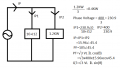# three phase star connected balanced supply question

#### B.Anderson

Joined Oct 25, 2016
1
Hi,

my teacher gave me this question and these answers but i don't understand how he got some of these answers i tried answering it myself and following the steps and using some of my notes but the notes are somewhat lacking in how to do this problem if someone could please guide me to understanding these answers and how to get them that would be great thanks!

A three phase star connected balanced supply which has 400 V, 50 Hz line voltage feeds a balanced star connected load consisting of;
(i) Three equal single phase loads of (10+j12) Ω
and
(ii) Three phase heating loads (purely resistive) of 1.2 kW

Calculate,
(a) Supply current
(b) Supply power factor
(c) Active and reactive power supplied
(d) Value of the capacitance that must be connected in star to improve the overall power factor to 0.95 lagging

the workingparts i dont understand:
how to get IP i tried calculating it myself and i dont get 15.96∠-45.4 (also why is the angle negative) i get 16.51∠50.194
how to get the pf(power factor)
how to get capacitance

#### MrAl

Joined Jun 17, 2014
10,101
Hi,

What are you calling the phase voltage?
Is it the line to line voltage or the phase to neutral voltage?

Do you know how you use complex numbers?

Power factor is cos(angle) where angle is the angle between current and voltage.

#### Dodgydave

Joined Jun 22, 2012
10,831
Ip2 will be phase to Neutral voltage of 230.9V, @1200W resistive, thats 5.19A, pf 1.